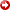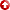[First Hit]Impact of Archie "m" on Evaluating Conventional and Low Resistivity Pay

R.M. Sneider, H. R. King, K. K. Stolper, J. T. Kulha

Correct interpretation of water saturation of many clastic reservoirs requires reliable estimates of Archie's lithology exponent "m". Archie "m" is related to formation factor (F), the ratio of the electrical resistivity of a 100% water saturated porous rock (R0) to the resistivity of the formation water (Rw). Archie "m" values are a function of (1) mineralogy including clay type, amount and distribution, (2) cementation and compaction, and (3) pore geometry (grain roughness, surface area, vugs, and pore-size distribution).

Petrophysical and petrological studies of thousands of reservoir rocks in many basins throughout the world show that "m" varies typically from 1.4 to 2.9. "m" is assumed to be 2 in many equations and log evaluation tables. If "m" is assumed to be 2, but the actual value is lower, hydrocarbon saturation calculates too low. Conversely, if "m" is assumed to be 2, but the actual value is higher than 2, the value of the hydrocarbon saturation is calculated too high.

The "m" value of many low resistivity, low contrast reservoir pays range from 1.5 to 1.8. Using "m" equal to 2, many pay zones appear "wet" or to have very high water saturation.

Log-derived water saturations using the correct "m" values versus "m" equal 2 are compared for several conventional and low resistivity pay zones. The use of rock comparators to estimate Archie "m" is illustrated.

AAPG Search and Discovery Article #91020©1995 AAPG Annual Convention, Houston, Texas, May 5-8, 1995